Next: Change of Binary Parameters: Up: Evolutionary Scenarios of Binary Previous: Wolf-Rayet and He stars

## Stellar Winds From Normal Stars

Ā

The effect of the normal star on the compact magnetized component is largely determined by the rateand velocity of stellar wind at infinity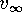. For the majority of cases, we assume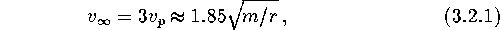whereis the parabolic velocity at the stellar surface.

For Be-stars (i.e. those stars at the stage ``I'' whose mass increased during the first mass exchange), the wind velocity at infinity is taken to be equal to the Keplerian velocityĀ at the stellar surface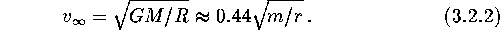Thus, the lower stellar wind velocity leads to an effective increase of the captured mass rate by a secondary companion to such Be-stars.

The stellar wind rate at stage ``I'' is calculated as (de Jager 1980)Hereis a numerical coefficient.

For giant post-MS stars (stage ``II''), we assumeand for massive star we take maximum wind rate between that given by de Jager's formula and that given by Lamers (1981):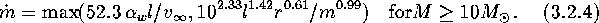For red supergiants at the asymptotic giant branch (AGB) stage, we use Reimers's formula (Kudritzki and Reimers, 1978)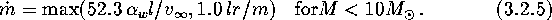When a massive star becomes a Wolf-Rayet star, its stellar wind can significantly increase (up to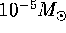yr). We parametrize such a windĀ aswhere the numerical coefficient is taken to be.

Mike E. Prokhorov
Sat Feb 22 18:38:13 MSK 1997